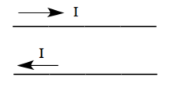# Problem: Two long straight parallel wires carry current in opposite directions, as shown in the sketch. The force that one wire exerts on the other is A) repulsive B) attractive C) zero

79% (76 ratings)
###### Problem Details

Two long straight parallel wires carry current in opposite directions, as shown in the sketch. The force that one wire exerts on the other is

A) repulsive

B) attractive

C) zeroFrequently Asked Questions

What scientific concept do you need to know in order to solve this problem?

Our tutors have indicated that to solve this problem you will need to apply the Magnetic Force Between Parallel Currents concept. You can view video lessons to learn Magnetic Force Between Parallel Currents. Or if you need more Magnetic Force Between Parallel Currents practice, you can also practice Magnetic Force Between Parallel Currents practice problems.

How long does this problem take to solve?

Our expert Physics tutor, Juan took 3 minutes and 14 seconds to solve this problem. You can follow their steps in the video explanation above.

What professor is this problem relevant for?

Based on our data, we think this problem is relevant for Professor Fink's class at TEXAS.